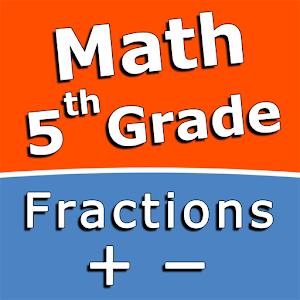Français

Chaque jour nous offrons des appli et jeux Androïdes GRATUITES que vous devrez autrement acheter.\$2.99
EXPIRÉ

# Android Giveaway of the Day - Add and subtract fractions - 5th grade math skills

Practice and improve the math skills!
\$2.99 EXPIRÉ
Note des utilisateurs: 1 (100%) 0 (0%)

This giveaway offer has been expired. Add and subtract fractions - 5th grade math skills is now available on the regular basis.

Intuitive interface powered by handwriting input and a choice of three fun and engaging mini games in addition to a regular math trainer mode make our app stand out from the crowd of generic math learning apps.

With Add and subtract fractions - 5th grade math skills you can practice and improve the following math skills:
- Add fractions with like denominators
- Subtract fractions with like denominators
- Add mixed numbers with like denominators
- Subtract mixed numbers with like denominators
- Add fractions with unlike denominators
- Subtract fractions with unlike denominators
- Add mixed numbers with unlike denominators
- Subtract mixed numbers with unlike denominators

Choose between four different modes:
- Math Trainer
- Math Shot mini-game
- Math Gravity mini-game
- Math Trick Shot mini-game

Sergey Malugin

Education

1.0.0

11M

Everyone

4.0 and up

## Commentaires sur Add and subtract fractions - 5th grade math skills

Thank you for voting!
Please add a comment explaining the reason behind your vote.
Windows Giveaway of the Day\$29.95
free today
100% clone data from one hard drive to another.